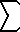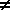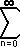Dave's Math Tables: Geometric Summations(Math | Calculus | Expansions | Series | Geometric)

 Summation Expansion Convergence Comments n-1r n n=0 = 1 + r + r 2 + r 3 + .. + r n-1 (first n terms) for r1, = ( 1 - r n ) / (1 - r) for r = 1, = nr Finite Geometric Seriesr n = 1 + r + r 2 + r 3 + ... for |r| < 1, converges to 1 / (1 - r) for |r| >= 1 diverges Infinite Geometric Series